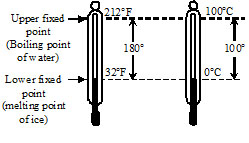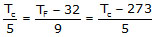# INTRODUCTION

Heat : It is a form of energy which causes the sensation of hotness or coldness.
For example, if we dip our finger in hot water we have a sensation of hotness. Similarly, If we touch a block of ice the sensation is that of coldness. In the former case the heat energy has moved into the      finger while in the later case it has moved out of the finger. Thus hotness or coldness basically indicates whether heat energy is flowing into our body or out of it.
Temperature :  It is the effect of heat energy which determines the thermal state of a given substance. In other words it determines the degree of hotness or coldness of a substance. If a body is at a higher        temperature than its surroundings, it means that heat energy will flow out of the body. Similarly, if a body is at a lower temperature than its surroundings, it means that heat energy will flow into the body.
Measurement of temperature: The instrument used for the measurement of temperature is called thermometer.

### DIFFERENT SCALES OF TEMPERATURE

(A) Celsius or centigrade scale :

As the name suggests, this scale has 100 divisions between the upper and lower standard points. This scale was introduced by a Swedish astronomer Celsius and is known after his name. On this scale 0°C represent melting  point of ice & 100°C represent steam point. Each division on this scale is called one degree centigrade or one degree Celsius and is written as °C.

(B) Fahrenheit Scale :

The scale was introduced by Fahrenheit. On this scale 32°F represents the melting point ofice and 212°F the steam point . Zero is marked 32°F below the ice point. The length in between the standard points is divided into 180 equal parts. Each division on this scale is called 1°F. This scale is widely used for meteorological and clinical purposes.

Relation between celsius and fahrenheit scales(C) Kelvin Scale

The scale of measurement of temperature, in which lowest temperature is zero Kelvin (–273°C) is called Kelvin scale. Thus is also called S.I. scale of temperature.Temp. in Kelvin = 273.15 + temp in °CCharacteristics of Kelvin scale

(i)    There cannot be any temperature below zero Kelvin
(i